# Replace Values of pandas DataFrame in Python (4 Examples)

In this Python tutorial you’ll learn how to exchange values in a pandas DataFrame.

The tutorial will contain this:

Let’s dig in…

## Example Data & Libraries

We first have to load the pandas library:

`import pandas as pd                                      # Import pandas library in Python`

Furthermore, consider the example data below:

```data = pd.DataFrame({'x1':range(1, 5),                  # Create example DataFrame
'x2':range(5, 1, - 1),
'x3':range(3, 7)})
print(data)                                             # Print example DataFrame```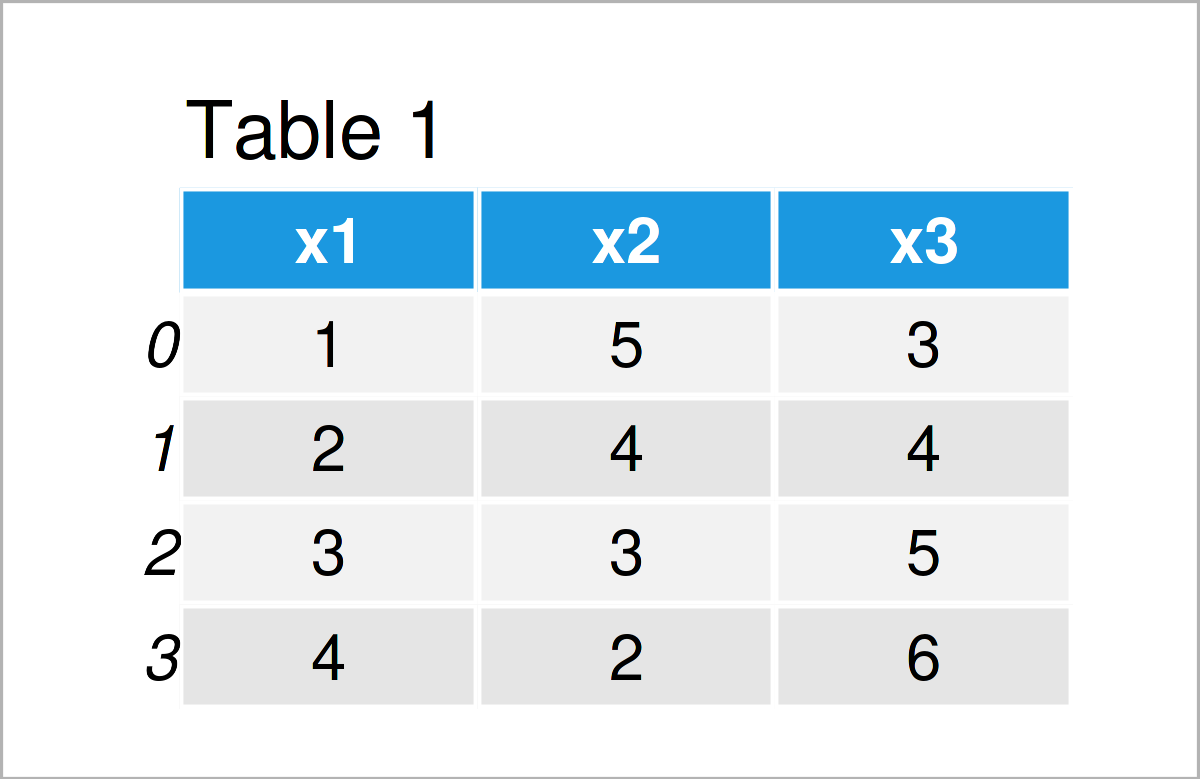As you can see based on Table 1, our example data is a DataFrame constituted of four rows and three variables.

## Example 1: Set Values in pandas DataFrame by Row Index

Example 1 demonstrates how to replace values in a certain pandas DataFrame column based on a row index position.

The following Python code creates a copy of our input DataFrame called data_new1, exchanges the DataFrame cell at the second row index position of the variable x1 by the value 999, and prints the output to the console:

```data_new1 = data.copy()                                 # Create copy of DataFrame
data_new1.at[2, 'x1'] = 999                             # Replace values in DataFrame
print(data_new1)                                        # Print updated DataFrame```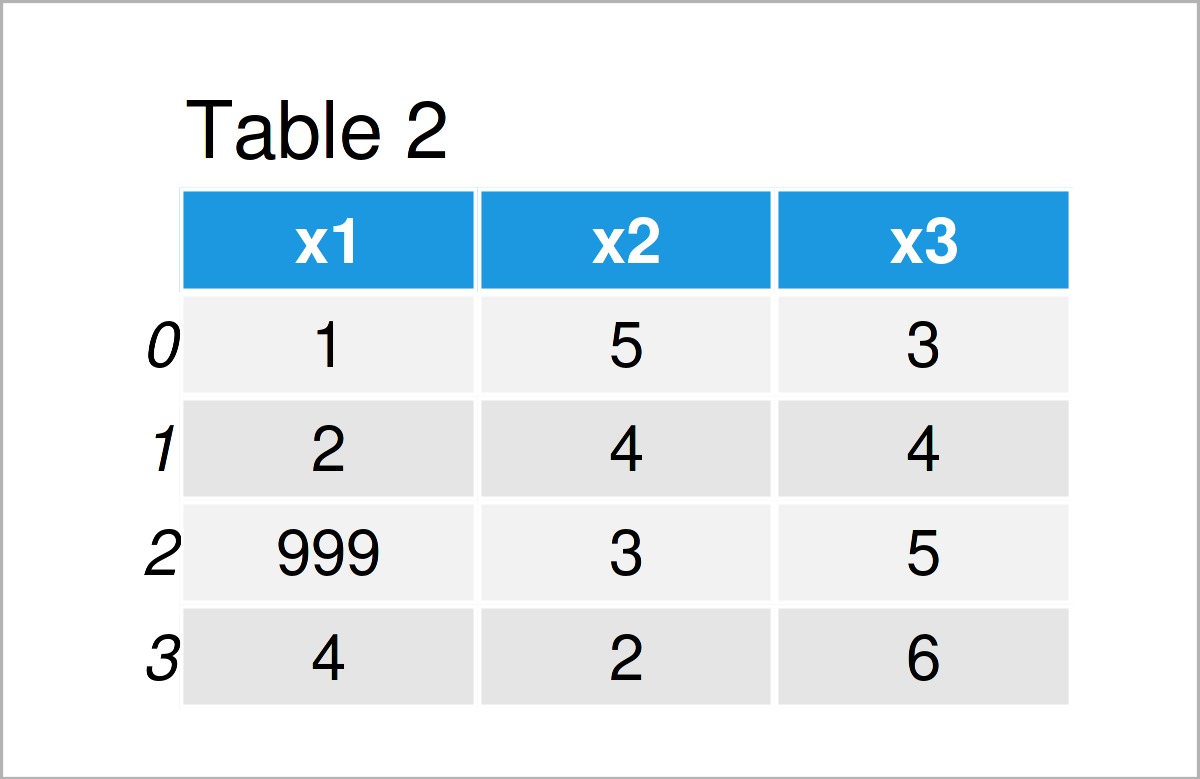As shown in Table 2, the previously illustrated Python programming syntax has created a new pandas DataFrame, in which a specific data cell has been substituted by a new value.

## Example 2: Exchange Particular Values in Column of pandas DataFrame Using replace() Function

In this example, I’ll show how to replace specific values in a column by a new value.

For this task, we can use the replace() function as shown below:

```data_new2 = data.copy()                                 # Create copy of DataFrame
data_new2['x1'] = data_new2['x1'].replace([1, 3], 999)  # Replace values in DataFrame
print(data_new2)                                        # Print updated DataFrame```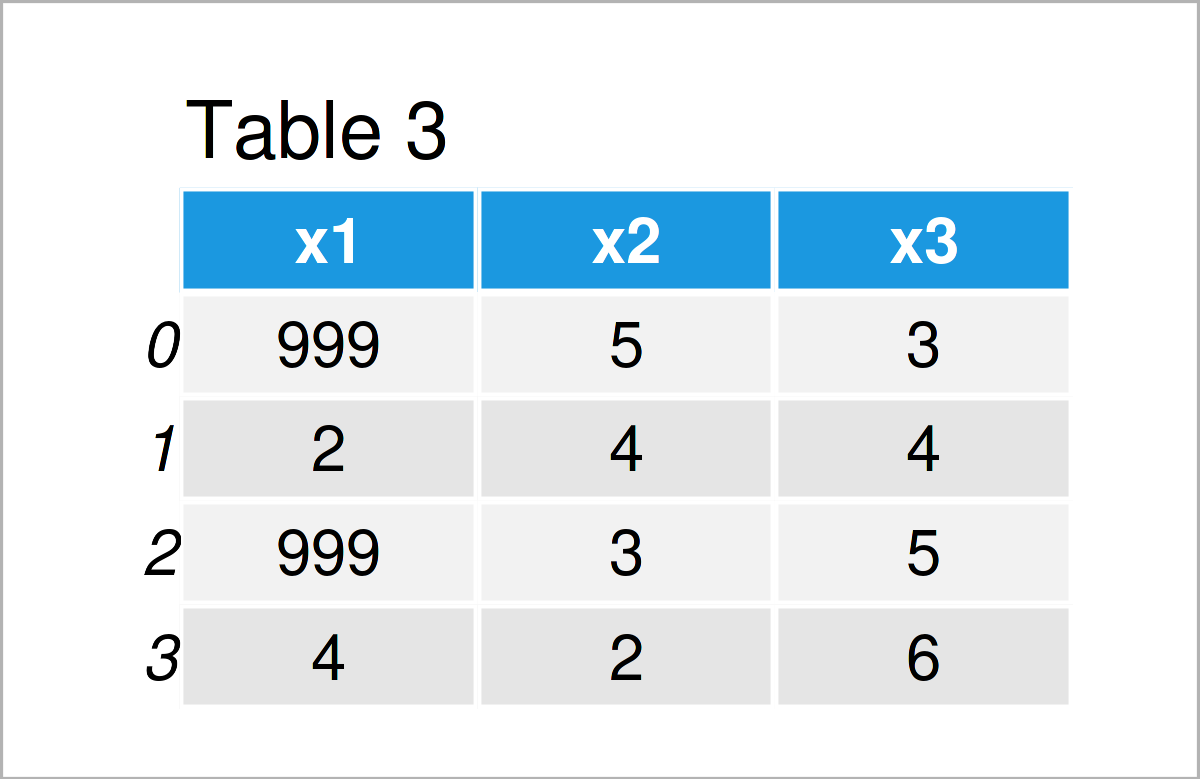As shown in Table 3, we have created another pandas DataFrame where the values 1 and 3 in the variable x1 have been replaced by the new value 999.

## Example 3: Exchange Particular Values in Entire pandas DataFrame Using replace() Function

Example 3 demonstrates how to substitute particular values by a new value in an entire pandas DataFrame (i.e. in all columns).

Consider the following Python syntax:

```data_new3 = data.copy()                                 # Create copy of DataFrame
data_new3 = data_new3.replace(4, 999)                   # Replace values in DataFrame
print(data_new3)                                        # Print updated DataFrame```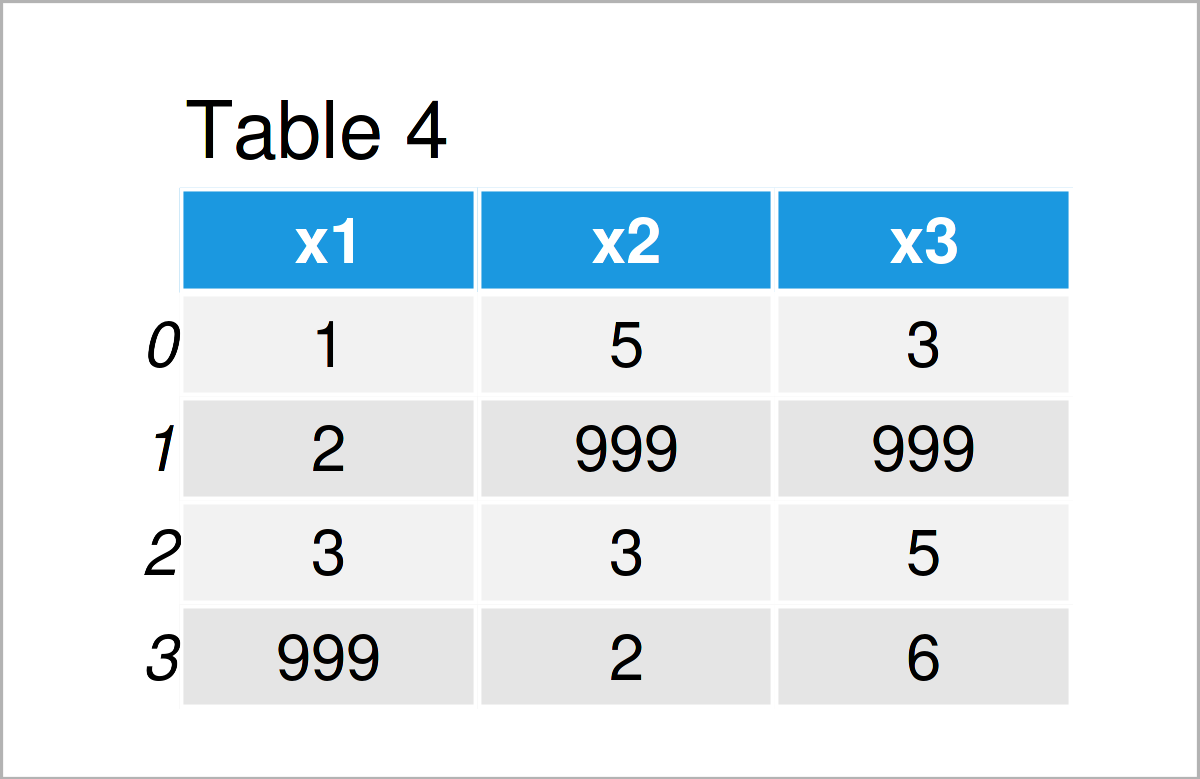After executing the previous Python programming code the pandas DataFrame shown in Table 4 has been created.

## Example 4: Replace Values in pandas DataFrame Conditionally

The following code demonstrates how to exchange cells in a pandas DataFrame according to a logical condition.

The Python code below replaces all values that are smaller or equal to 2 in the column x1 by the value 999:

```data_new4 = data.copy()                                 # Create copy of DataFrame
data_new4.loc[data_new4.x1 <= 2, 'x1'] = 999            # Replace values in DataFrame
print(data_new4)                                        # Print updated DataFrame```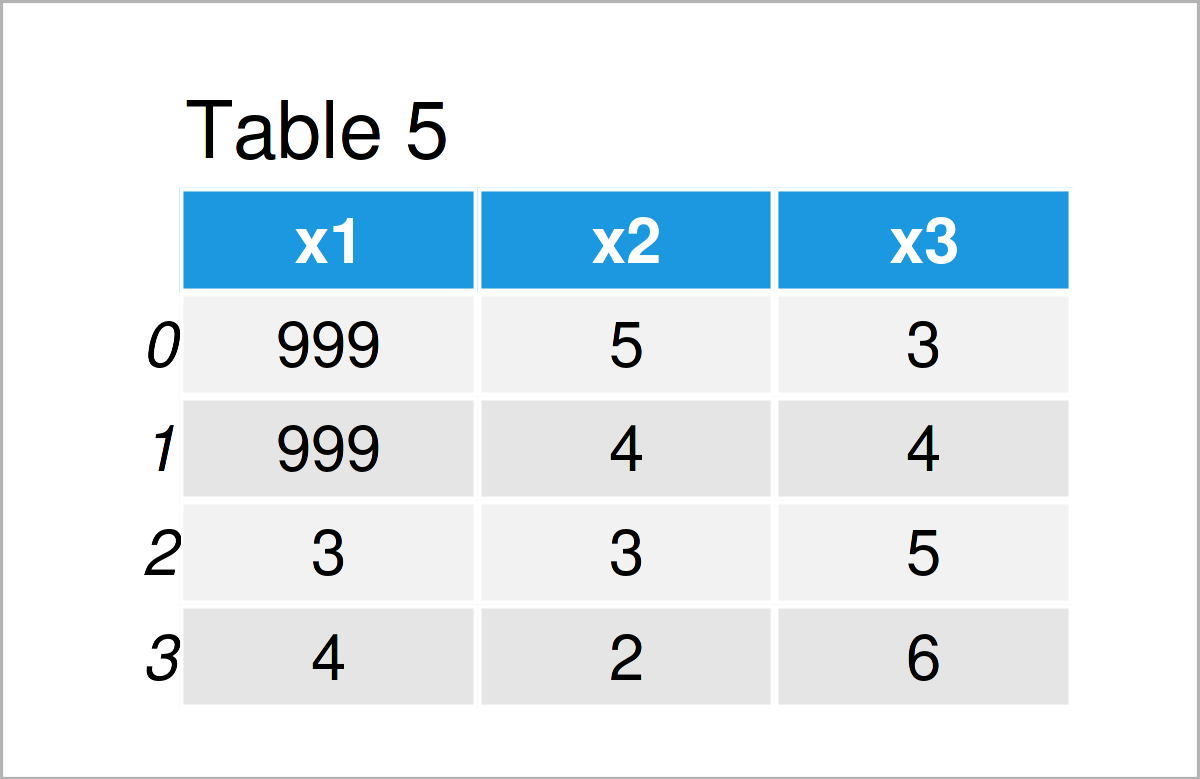After running the previous Python programming code the pandas DataFrame illustrated in Table 5 has been created.

## Video, Further Resources & Summary

Have a look at the following video which I have published on my YouTube channel. I show the examples of this tutorial and give some extra information.

Please accept YouTube cookies to play this video. By accepting you will be accessing content from YouTube, a service provided by an external third party.If you accept this notice, your choice will be saved and the page will refresh.

In case you need further explanations on the topic, I recommend having a look at the following video on the YouTube channel of Noureddin Sadawi. In the video, he shows additional examples for replacing values in a pandas DataFrame in Python:

Please accept YouTube cookies to play this video. By accepting you will be accessing content from YouTube, a service provided by an external third party.If you accept this notice, your choice will be saved and the page will refresh.

In addition, you might have a look at some of the related tutorials on my website. I have released numerous tutorials about the replacement of values in a pandas DataFrame already:

In this tutorial, I have explained how to replace values in a pandas DataFrame in the Python programming language. If you have further questions, please let me know in the comments section. Besides that, please subscribe to my email newsletter in order to get updates on new tutorials.

Subscribe to the Statistics Globe Newsletter